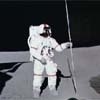#### You may also likeA circle is inscribed in an equilateral triangle. Smaller circles touch it and the sides of the triangle, the process continuing indefinitely. What is the sum of the areas of all the circles?### Lunar Leaper

Gravity on the Moon is about 1/6th that on the Earth. A pole-vaulter 2 metres tall can clear a 5 metres pole on the Earth. How high a pole could he clear on the Moon?### So Big

One side of a triangle is divided into segments of length a and b by the inscribed circle, with radius r. Prove that the area is: abr(a+b)/ab-r^2

# Can You Prove It?

##### Age 16 to 18Challenge Level

Here are a collection of statements for you to try to prove clearly and concisely. Once you have had a go, follow the links to see how other students solved each one.

Perfectly Square
We are first given that: $$x_1 = 2^2 + 3^2 + 6^2$$ $$x_2 = 3^2 + 4^2 + 12^2$$ $$x_3 = 4^2 + 5^2 + 20^2$$ Then show that $x_n$ is always a perfect square.

Always Perfect
Prove that if you add 1 to the product of four consecutive whole numbers the answer is ALWAYS a perfect square.

Unit Interval
Take any two numbers between $0$ and $1$. Prove that the sum of the numbers is always less than one plus their product.

That is, if $0< x< 1$ and $0< y< 1$ then prove

$$x+y< 1+xy$$.

Sums of Squares
$2(5^2 + 3^2) = 2(25 + 9) = 68 = 64 + 4 = 8^2 + 2^2$
$2(7^2 + 4^2) = 2(49 + 16) = 130 = 121 + 9 = 11^2 + 3^2$
Prove that if you double the sum of two squares you get the sum of two squares.

Mod 3
Prove that if $a^2+b^2$ is a multiple of $3$ then both $a$ and $b$ are multiples of $3$.

IFFY Triangles

1. Let $ABC$ be a triangle with angles $A$, $B$ and $C$ where $A \le B \le C$ and $A$, $B$ and $C$ form an arithmetic progression. Prove that $B=60^{\circ}$.

2. $XYZ$ is a triangle with angles $X$, $Y$ and $Z$ and we have $Y = 60^{\circ}$.
Prove that $X$, $Y$ and $Z$ form an arithmetic progression.

3. $PQR$ is a triangle with length $PQ$ equal to length $QR$.
Prove that angles $P$ and $R$ are equal.

4. $KLM$ is a triangle with equal angles $K$ and $M$.
Prove that the lengths $KL$ and $LM$ are equal.